# The INDEX function

Uploaded time: October 28, 2021 Difficulty: Intermediate# The INDEX function

The INDEX function can look up a value regarding its row and column numbers.

There are two forms of the INDEX function, the Array form and the Reference form.

· One: Array form

We'll use the Array form to return the value located in the seventh row and the fourth column.

First, select cell J6. Next, click Formulas and then Insert Function to insert the INDEX function.

The function syntax:

INDEX(array,row_num,column_num)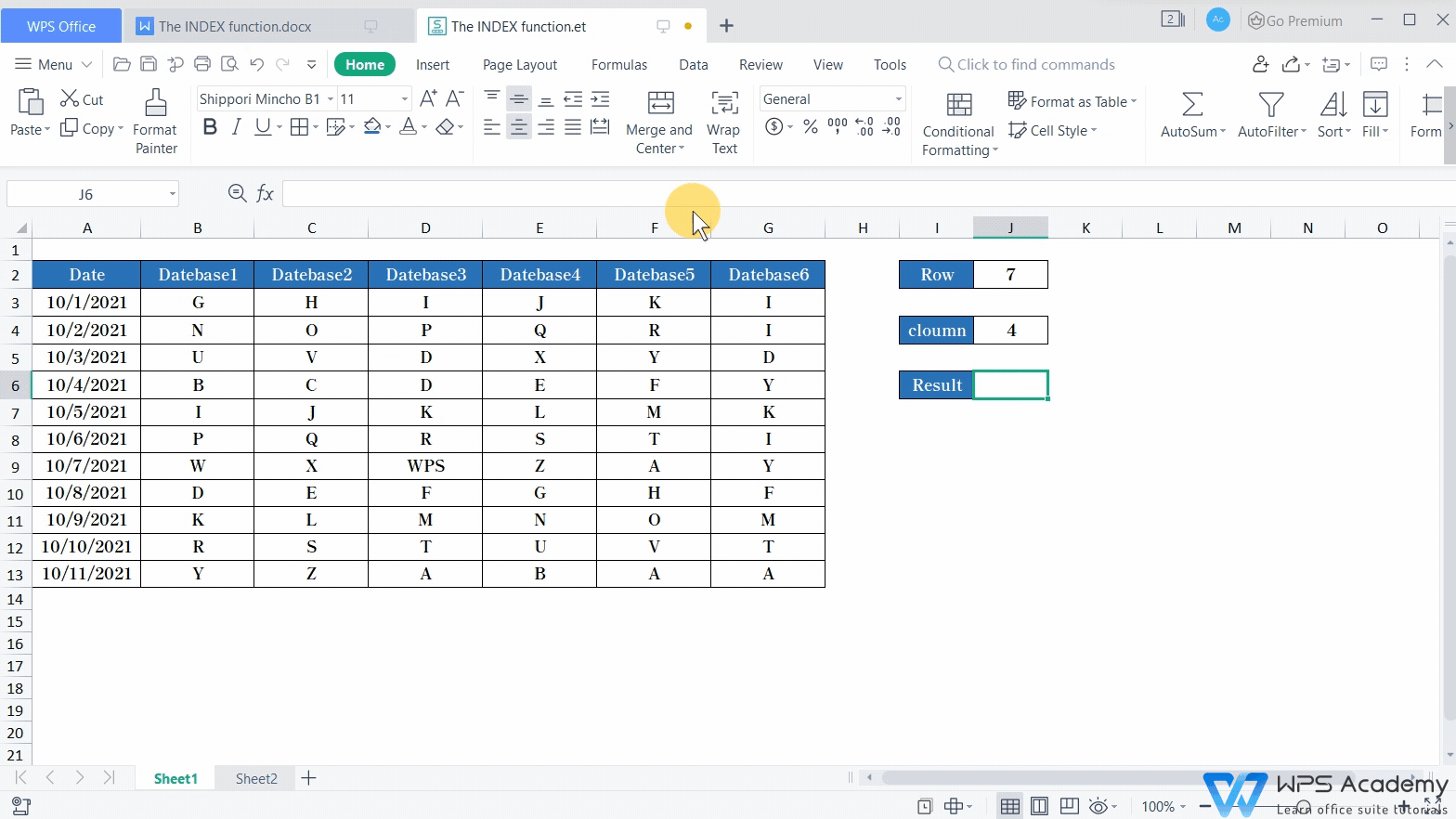The first parameter, 'Array', refers to the array for value lookup. Here we select A3:G13.

The second parameter, 'Row_num', refers to the row number of the value in the array. Here the row number is '7', so we enter '7' as the second parameter.

The third parameter, 'Column_num', refers to the column number. Here we've known that the column number is four, so we enter '4' as the third parameter.

In this way, we can get the lookup result 'WPS'.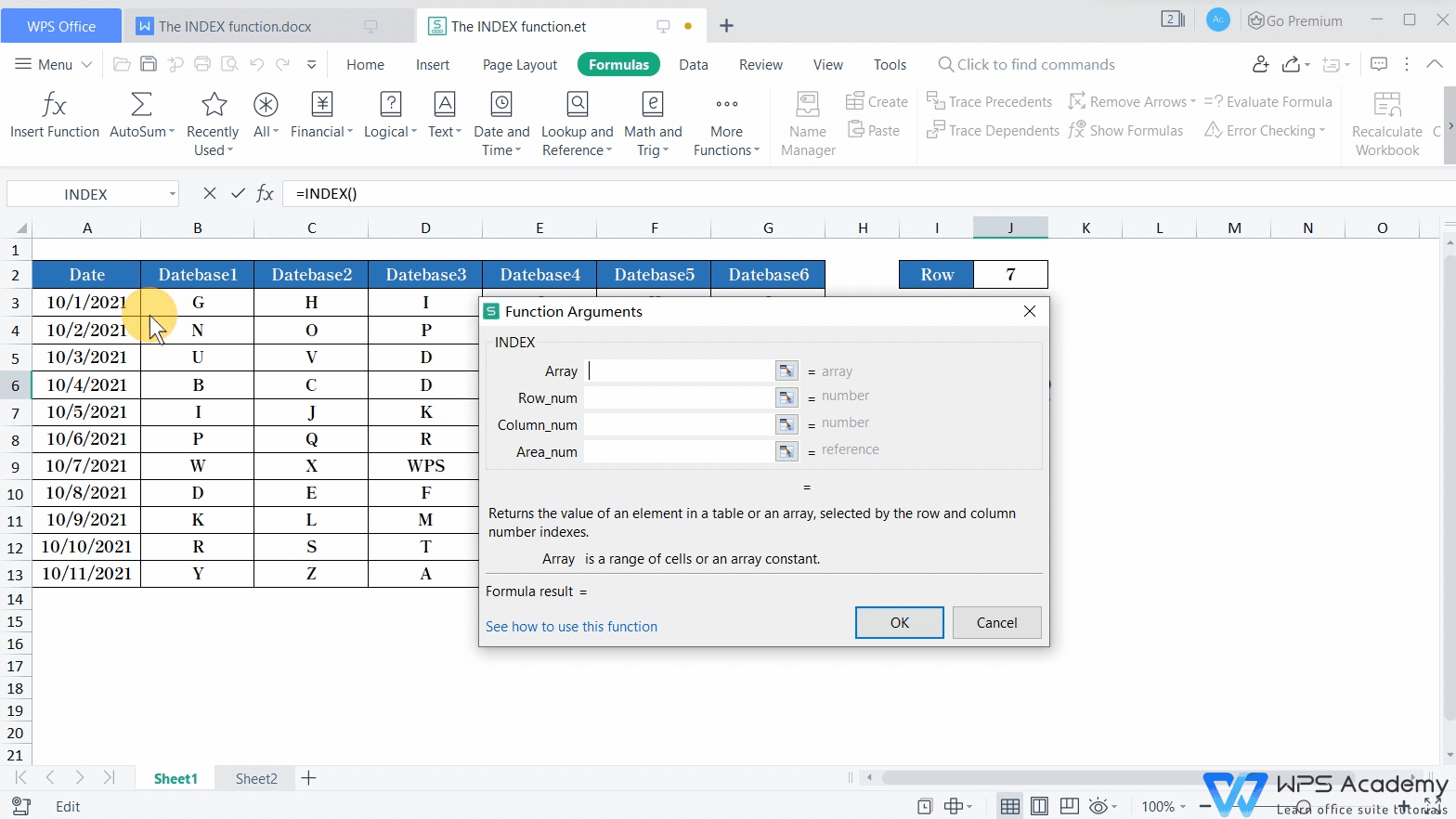· Two: Reference form

If we need to look up values among multiple incontinuous arrays, the Reference form of the INDEX function will also help.

In 'sheet2', statistics of four quarters are respectively presented in four arrays. For example, if we want to get the value located in the fifth row and third column of Q2, we can use the INDEX function.

The function syntax:

INDEX (Array,Row_num,Column_num,Area_num)

1. In the first parameter 'array', we select A3:C9,E3:G9,I3:K9,I13:K19. (* Note that parentheses are needed.)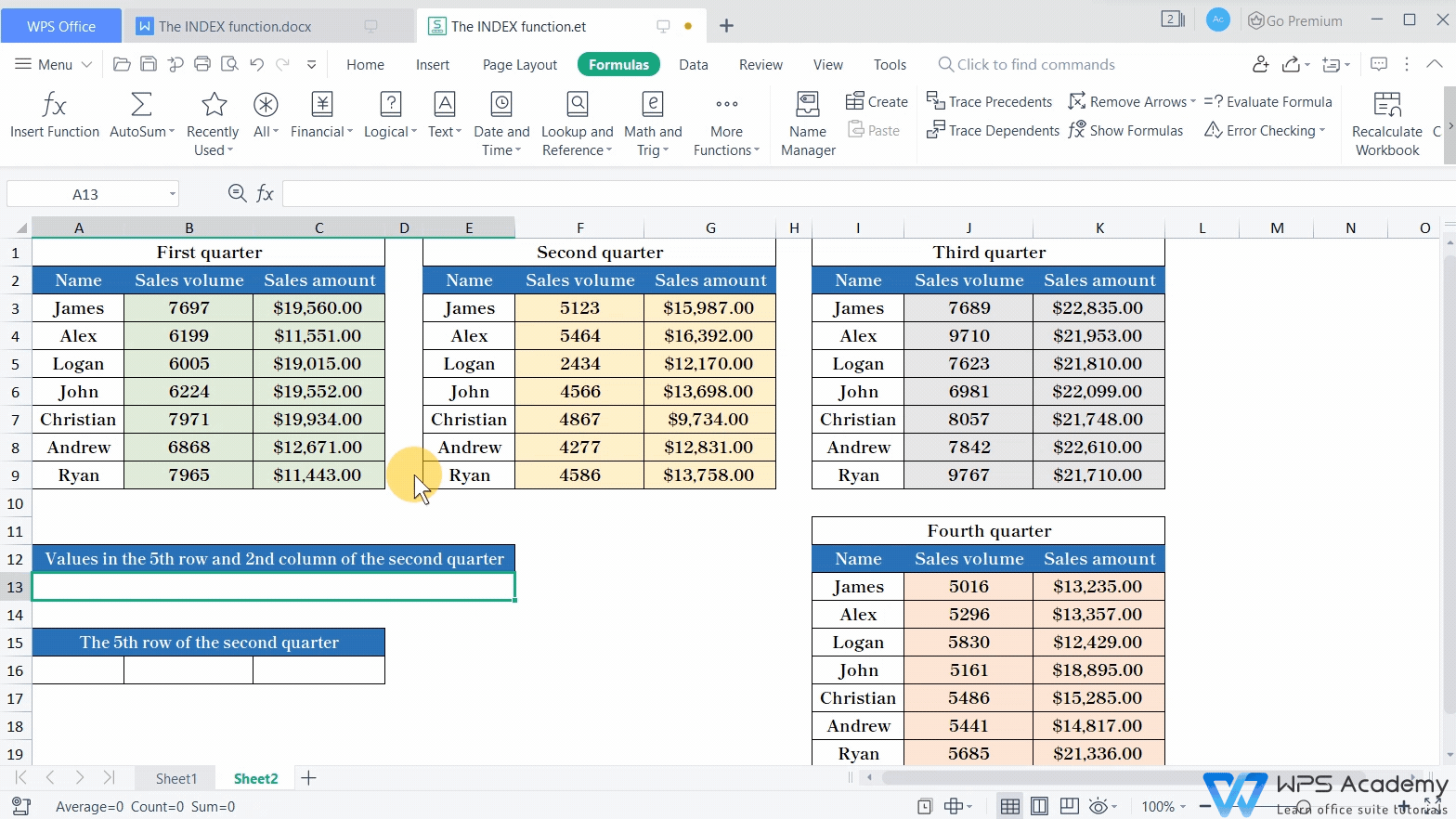2. Enter '5' as the second parameter.

3. Enter '3' as the third parameter.

4. The fourth parameter, 'Area_num', refers to the array for value lookup.

Take the first parameter as an example. A3:C9 refers to array 'Q1'. E3:G9 refers to array 'Q2'. I3:K9 refers to array 'Q3'. I13:K19 refers to array 'Q4'.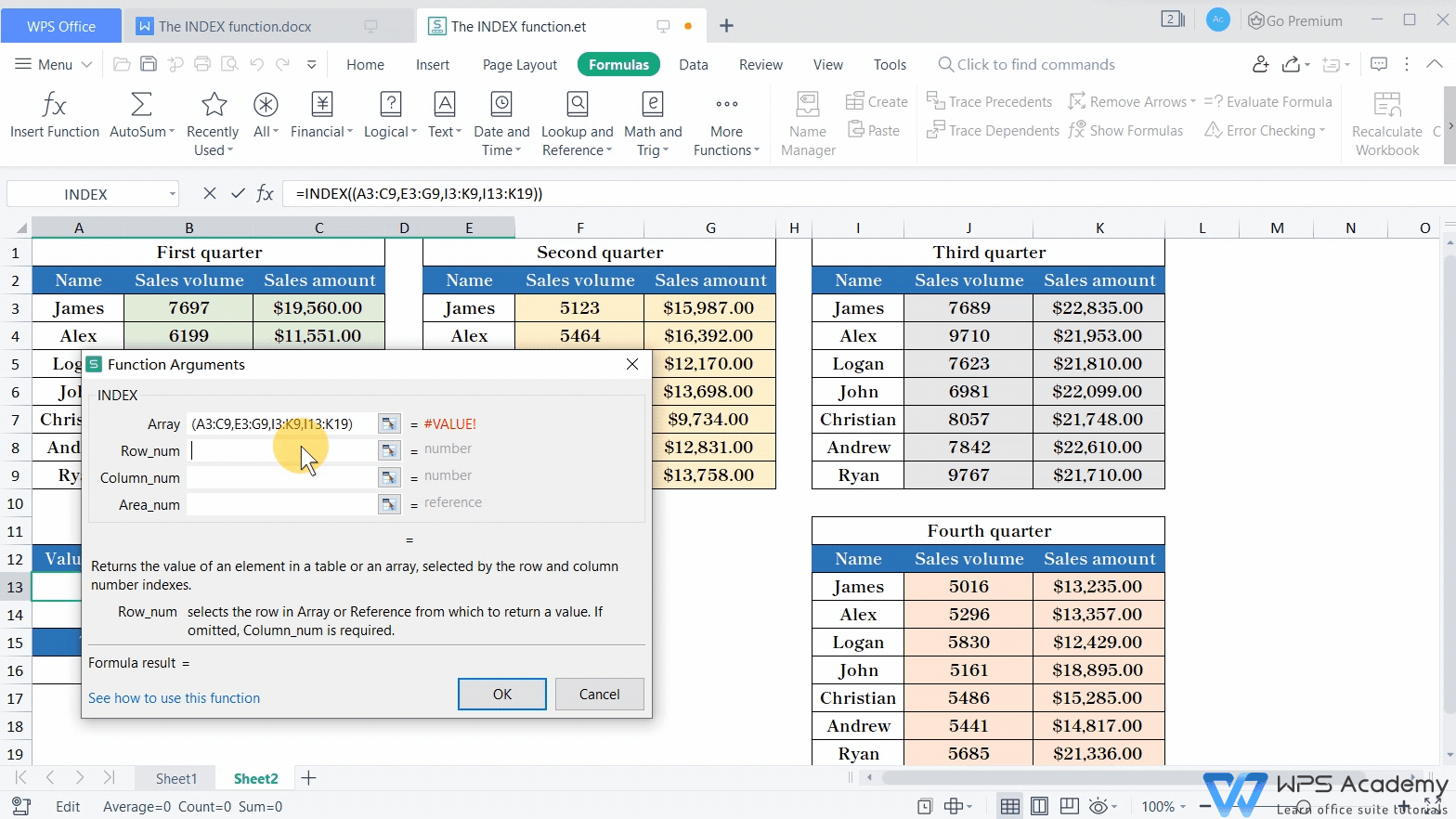Suppose that the value we want to find is from Q1, then enter '1' as the fourth parameter.

If we want to find the value from Q3, enter '3' as the fourth paramater.

The INDEX function allows us to look up values from various arrays when processing lots of data.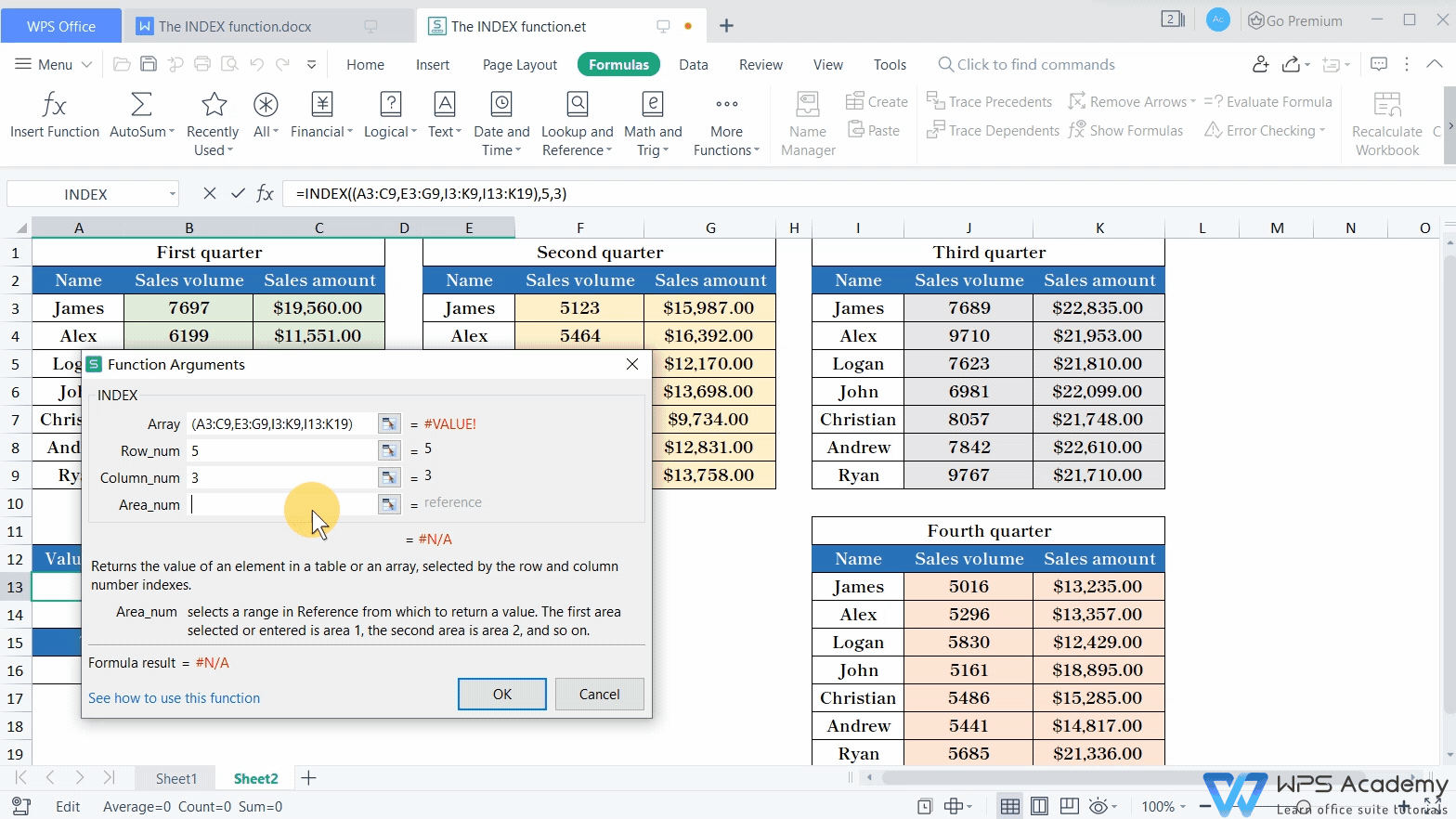In the previous part, we've learned how to return a single value with INDEX. The INDEX function can also return a whole row or column of data.

For example, if we want to return the fifth-row data of Q2, select cell A16:C16 and enter the function:

=INDEX((A3:C9,E3:G9,I3:K9,I13:K19),5,,2).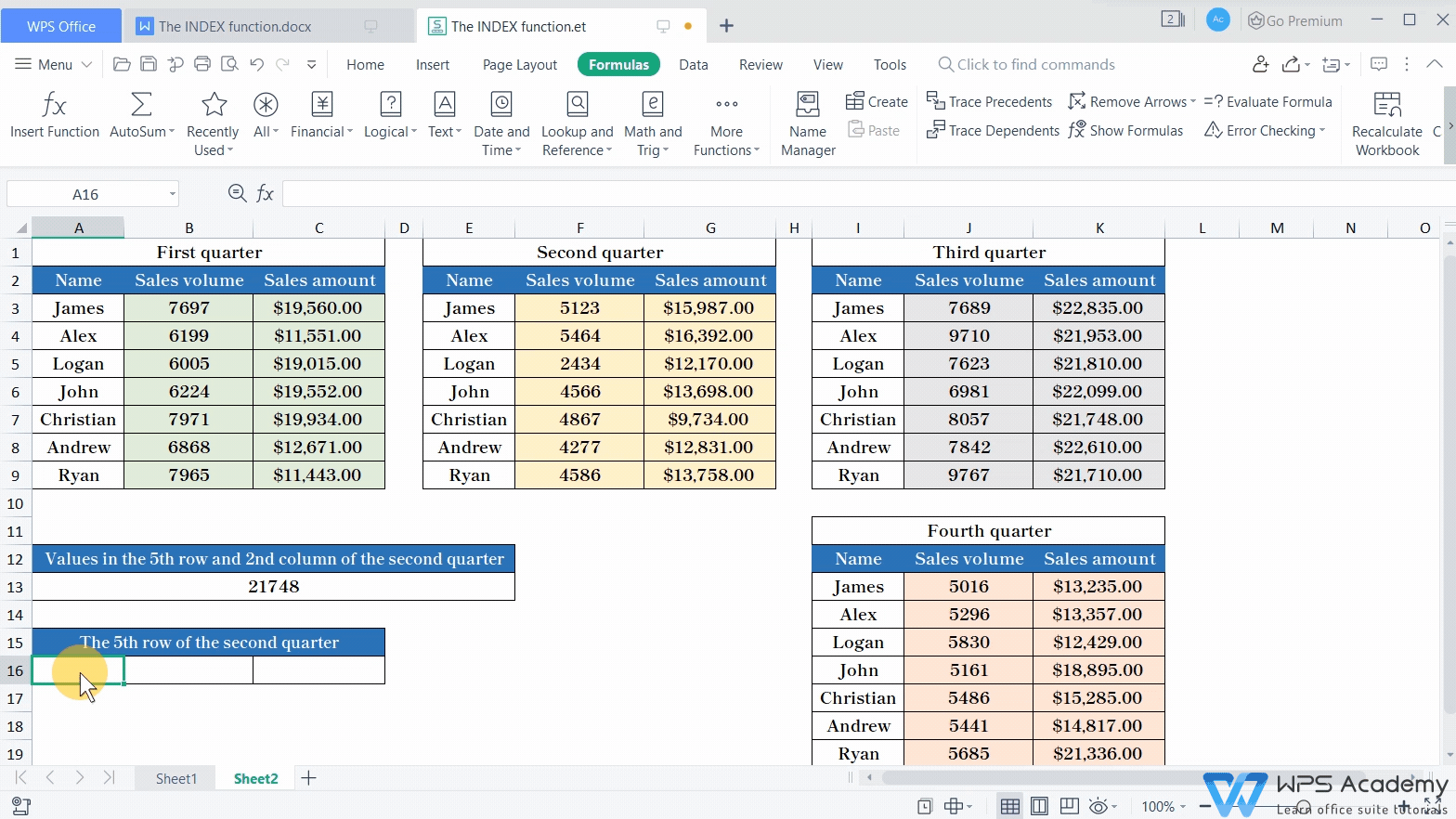If we want to return all values in the whole fifth row, enter '5' as the second parameter and leave the third parameter blank. Press Enter. The result will be 'ERROR'.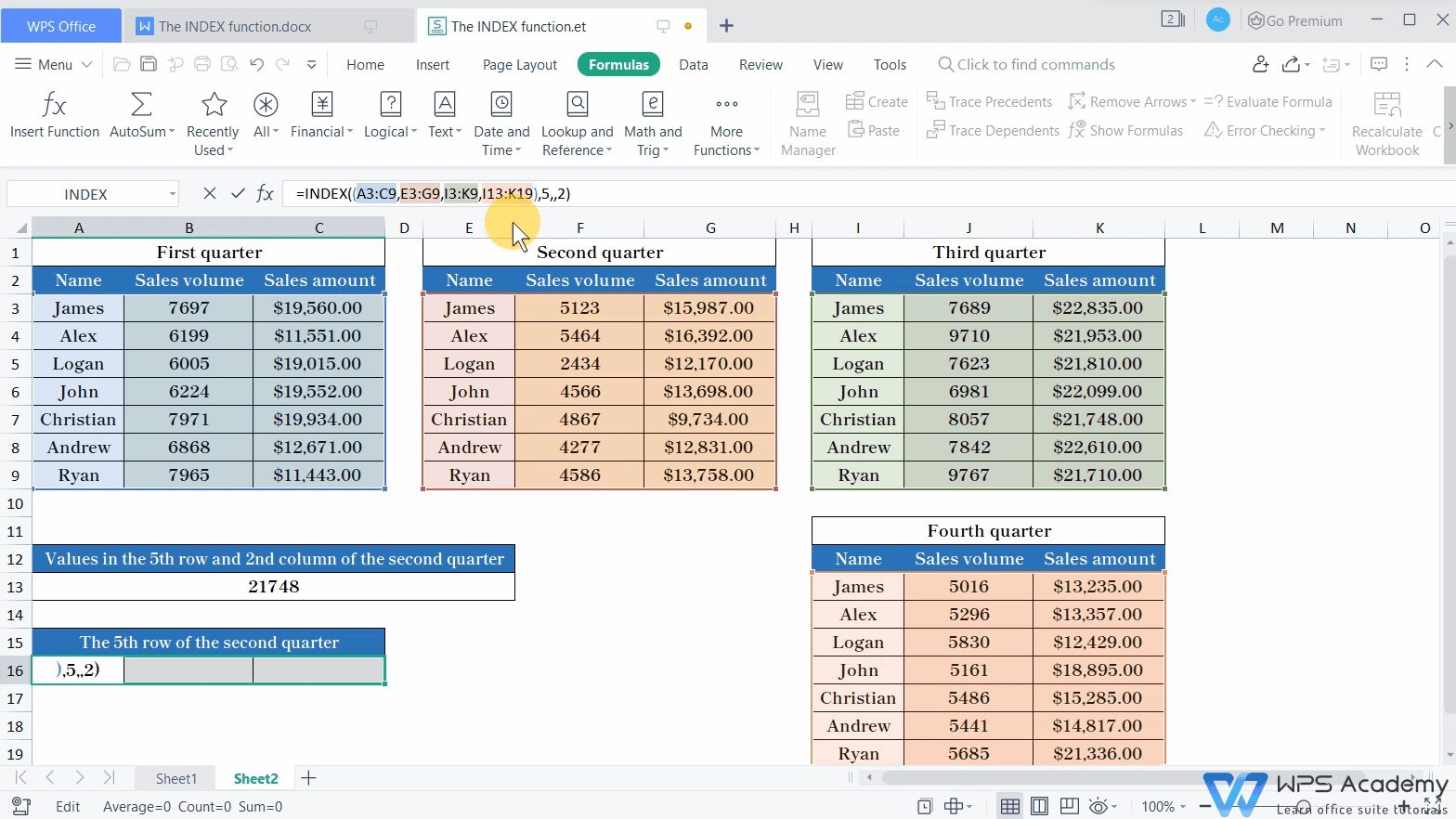As INDEX is an array formula, parentheses are needed to enclose the array formula. To fix the problem, select cell A16:C16 and press Ctrl+Shift+Enter. Then, we'll get the expected result.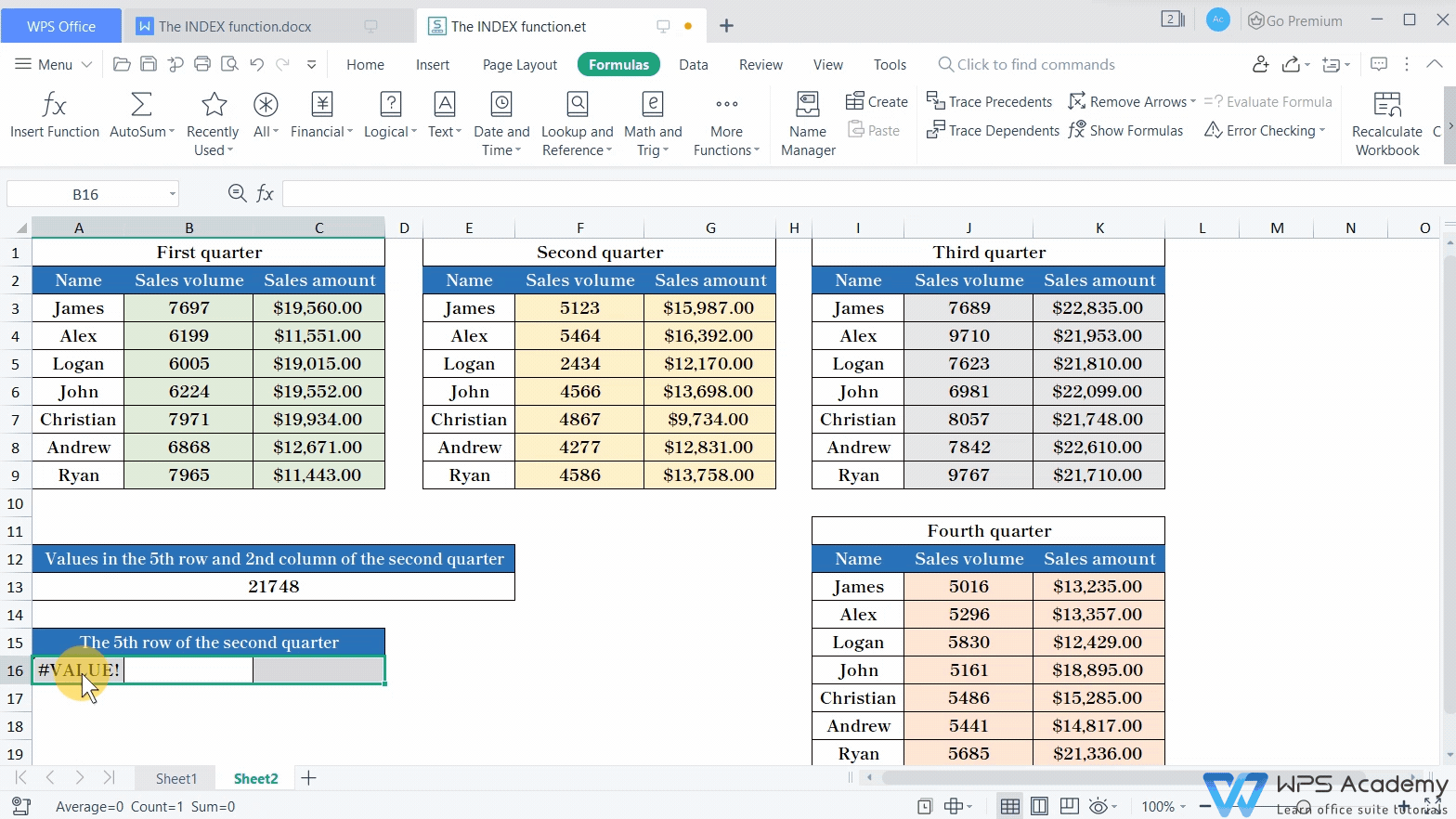Here comes to the end of the tutorial. Did you get it?